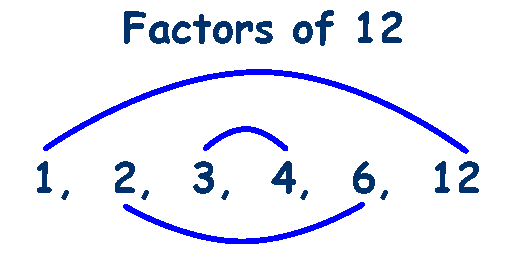# Math 10 Week 10

This week I learned how to factor a simple polynomial. A simple polynomial means the leading coefficient is a one. To factor a simple polynomial you need to know what factoring is. Factoring is finding all the different numbers that multiply to get a certain number. Ex. 24 the factors of 24 are 1,2,3,4,6,8,12,24. Now I can teach you how to factor a simple polynomial.

Ex.$x^2$ + 8x +12

Step one: Factor the constant.Step Two : Look at the clues; which of the pairs subtract or add to give you the middle term.

In this case it would be 2 and 6. Another clue is that everything is positive so the factors will be positive.

Step Three: Start writing it out

*You know that x times x is$x^2$ so the x’s will go at the front of the brackets. (x___)(x___)

The answer is (x +2) (x +6)